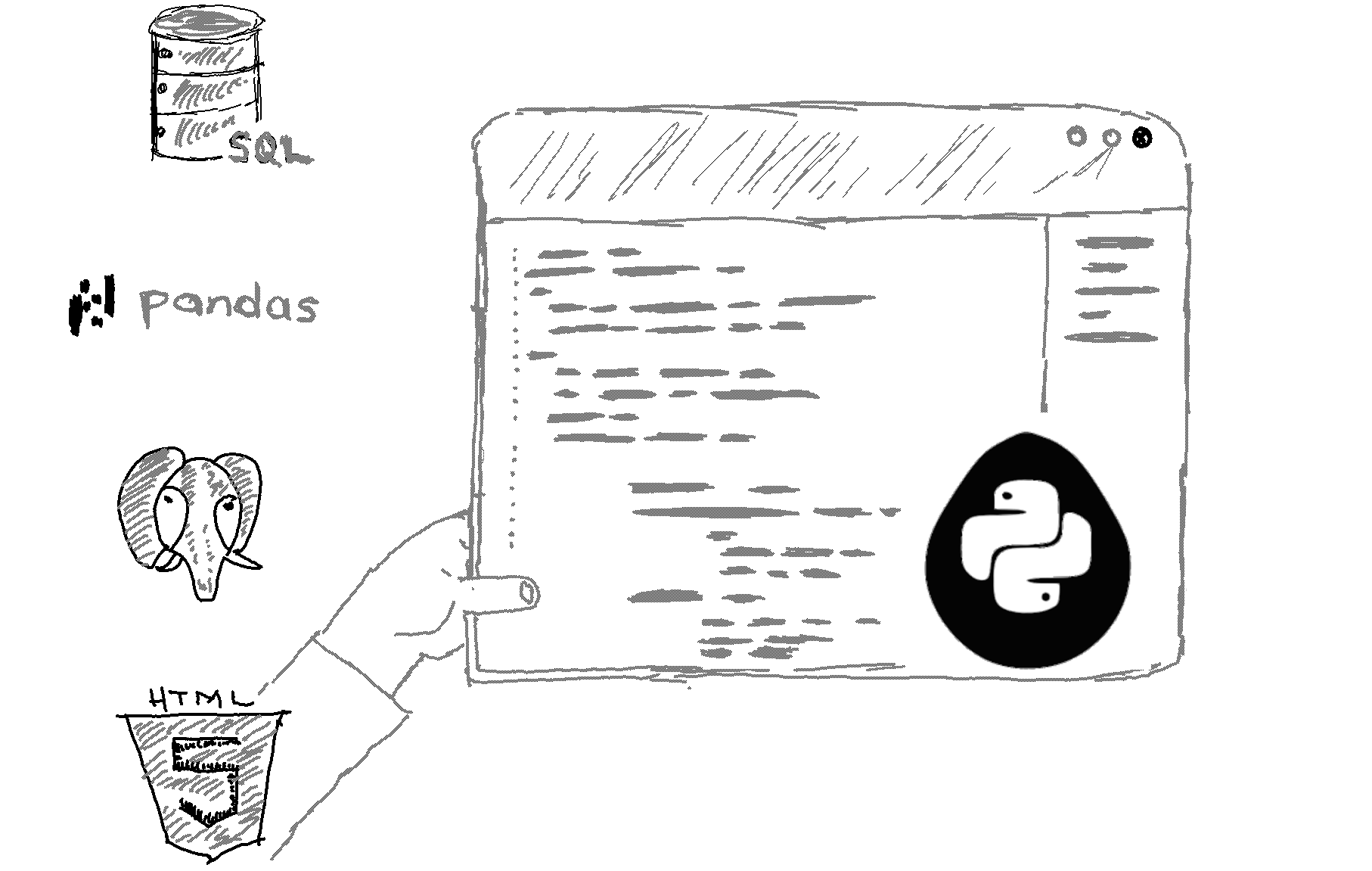# Here is how to delete a column from a pandas dataframe in Python.

```# Import the library and create a dataframe object
import pandas
df = pandas.DataFrame({'A': [1, 2], 'B': [10, 20]}) ```
```# Delete column A
del df['A'] ```
```# Print out the updated dataframe
print(df) ```

Output

B

0  10

1  20

Explanation

The del command can be used to delete a pandas dataframe column.

Another alternative that would also delete a dataframe column is this:

df = df.drop('A', axis=1)

Note that drop does not modify the original dataframe. Instead, it returns a new modified dataframe object. In the line above, we are reassigning that new object to the existing df variable.

Related HowTos
##### Recommended Course

Learn Flask development and learn to build cool apps with our premium Python course on Udemy.